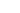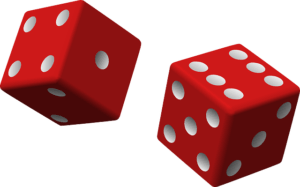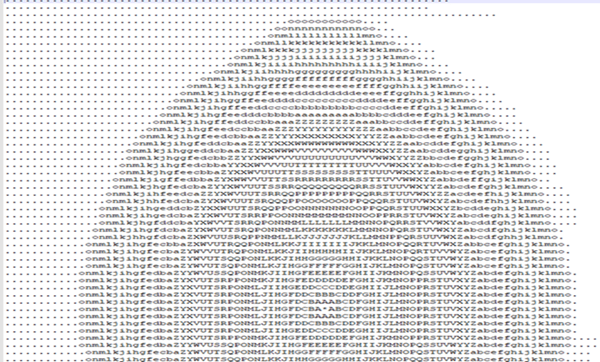### Tag: random## A fast random number generator in CIf you are using RNGs (Random Number Generators) for cryptography then you need one that has been validated for sufficient randomness. For example the libsodium library.

But if you want a fast one for general purpose use, then xoshiro256++ is a fast one.  It’s actually that short that I can list it here. Just call next() and mod it (%) with the highest value+1. E.G. for a dice roll,

``````int diceroll= (next() %6) +1;
``````

This algorithm is very fast, typically on the order of nano-seconds 10-9 seconds.

``````#include <stdint.h>
uint64_t rngstate;

static inline uint64_t rotl(const uint64_t x, int k) {
return (x << k) | (x >> (64 - k));
}

// Returns a Uint64 random number
uint64_t next(void) {
const uint64_t result = rotl(rngstate + rngstate, 23) + rngstate;
const uint64_t t = rngstate << 17;
rngstate ^= rngstate;
rngstate ^= rngstate;
rngstate ^= rngstate;
rngstate ^= rngstate;
rngstate ^= t;
rngstate = rotl(rngstate, 45);
return result;
}``````## More on generating random numbersImage by Clker-Free-Vector-Images from Pixabay

The very first program I wrote in any programming language was sometime in October 1976. It was in BASIC and it had this line or something very similar. Even then I was into random numbers. I know, uppercase!

``LET DIEROLL = RANDOM(6)+1``

I’ve been writing small utility that I’ll publish in a few days. It’s 100% in C and I decided instead of using srand(time(null)) for generating random numbers, I’d use something with a bit more oomph. That something is a library called sodium. Using it for just generating random numbers is a bit overkill. But it has the benefit that the random numbers are good enough random to be used in cryptographic code. Not all random number generators are good enough. This one is.

And it’s easy enough and adds just one dll, or you can compile it statically. I’ll do that once I figure it exactly which Visual Studio settings to change.

It takes just one include –

``#include <sodium.h>``

Then one call to initialise it.

`````` int i = sodium_init();
if (i<0 ) {
error("Unable to initialise sodium library. Error = ",inttoa(i) );
}``````

After that you can call one of several functions. Here’s an example from my code. It fills a 64 byte key with the numbers 0-63 but shuffled like cards.

``````
typedef char key;
key _key;
uint32_t ival;
int i,index1,index2;
for (i = 0; i < 63; i++) {
_key[i] = i;
}
for (i = 0; i < 1000; i++) {
do {
index1 = randombytes_uniform(64);
index2 = randombytes_uniform(64);
} while (index1 == index2);
ival = _key[index2]; // swap two indices
_key[index2] = _key[index1];
_key[index1] = ival;
}``````

The do loop (how often do you actually see those used in code!) generates two indexes in the range 0-63, making sure that they are not the same. It then swaps the two values at those indexes, and does this 1,000 times. It’s very similar to card deck shuffling code.

Do you know how long it would take you to work through all the combinations of 64 numbers this way? IF you could do a million a second, it would take you 1.27×1083 seconds or 4.02×1075 years! Or to make it more meaningful it’s this large! 4.02,000,000,000,000,000,000,000,000,000,000,000,000,000,000,000,000,000,000,000,000,000,000,000,000,000. That’s approaching (but still far far off) the time for the heat death of the universe which is estimated at 10100!## About that Empire Map GeneratorThis post refers to the c sources in the AboutEmpire.zip file in the Github C Games repository.

I first developed the algorithm in 1986 and wrote it in Z80 assembler. It was then rewritten in Turbo Pascal and used in both the Warlord and Quest postal games that are still run by kjcgames.com. It has been rewritten in C in the Empire game. So how does it work?

The idea is to generate continents. Typically between 2 and 3 continents between 1500 and 1800 squares in total on an 80 x 50 map. The map is made up of land squares and sea squares but starts out blank.

First I throw down 30 land points and 50 sea points. I.e. the program randomly picks points.

``````void AddPoints(enum loctype lt,int numpoints) {
int i,x,y;

for (i=0;i<numpoints;i++) {
do {
x=Random(MAPWIDTH-40)+20;
y=Random(MAPHEIGHT-40)+20;
}
while (map[x][y].locis != lctnull);
map[x][y].locis = lt;
genpointsx[pointindex]=x;
genpointsy[pointindex++]=y;
}
}``````

Some of the constants and the loctype enum are defined in common.h. The map itself is built up by adding extra points to each land point and sea point. I’ve defined an array of points in data.h that has 35 rings of points.  Well they’re more squares than rings.  That text file shown in the image is what these rings are derived from. I’ve translated all the points into 35 sets of X,Y offsets in data.h.

These points get added in layers. Starting with the first layer which has 8 points in it around the centre – that’s the A’s in the image, the next layer has 12 B’s and 4 C’s in it and so on.

But I only add a land or sea point if the spot its going into is empty; once it is defined as sea or land it can never change. So sea points and land points bump up against each other as these ‘circles’ expand and you get coasts and all sorts of interesting shapes. After that I fill in all empty spaces as sea, and the count up the connected land and sea squares.

If there are any tiny seas, i.e. less than 5 squares area they get filled in as land and any land point that isn’t surrounded by at least 3 other land point gets sunk. Then so long as there are the right number of continents and the total land squares is between 1500 and 1800, the map is accepted. If not a new one is generated. Adjusting the number of initial land and sea points helps to generate more acceptable maps.